## DIGITAL ELECTRONICS

electronics is concerned with straightforward two-state switching circuits. Traditional applications which have analogue inputs such as audio recordings are now using digital techniques, with the development of analogue-to-digital converters. These convert the analogue voltage into digital numbers.### COMPARISON OF ANALOGUE AND DIGITAL WAVEFORMS

The digital waveform has two quite definite states, either on or off, and changes between these two states very rapidly. An analogue waveform changes its value smoothly and progressively between two extremes.

In an analogue system, changes in component value due to ageing and temperature can affect the circuit’s performance. Digital systems are very much less susceptible to individual component changes.

Analogue circuitry is easily contaminated by noise particularly when signal levels are very small. Digital signals however, have very large amplitude and can be made relatively free of noise thereby achieving high quality of sound reproduction.

### LOGIC CIRCUITS

Logic circuits have been developed to deal with these digital two-state switching circuits. Information is expressed as binary numbers, that is, numbers which consist of ones and zeros. The low level is called logic 0 and the high-level logic 1. The information is processed according to rules built into circuits made up of signal units called logic gates.

#### LOGIC GATES

A logical gate performs a logical operation on one or more inputs and produces a single logical output.

A logical circuit is a two – position device with ON (HIGH) and OFF (LOW) state. Logic circuit behaves like a switch.

#### Basic Logic Gates

1) AND GATE

2) OR GATE

3) NOT GATE

4) NOR GATE

5) NAND GATE

6) XOR GATE

7) XNOR GATE

#### THE AND LOGIC GATE

The signal lamp will only illuminate if both switch A and B are closed. Alternatively, the output F of the gate will only be at logic 1 if both input A and B are both at logic 1.

If the AND gate was operating a car handbrake warning lamp, it would only illuminate when both the handbrake and the ignition were on.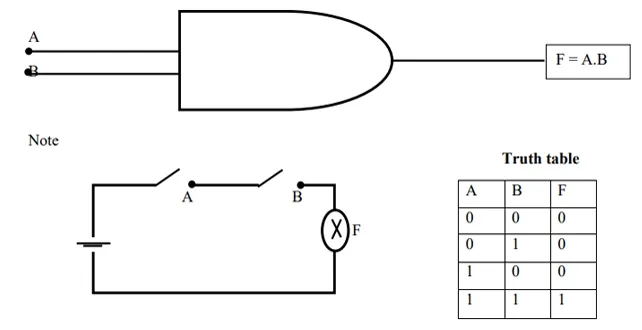Switch Arrangements equivalent to AND logic

The lamp F only lights when both switches are closed.

#### THE OR LOGIC GATE

The signal lamp will only illuminate if switch A or B or both switches are closed. We could also say that the output F will only be at logic 1 if input A or B or both inputs are at logic 1.

If the OR gate was operating an interior light in a motor car, it would illuminate when the nearside door was opened or the offside door was opened or when both doors are opened.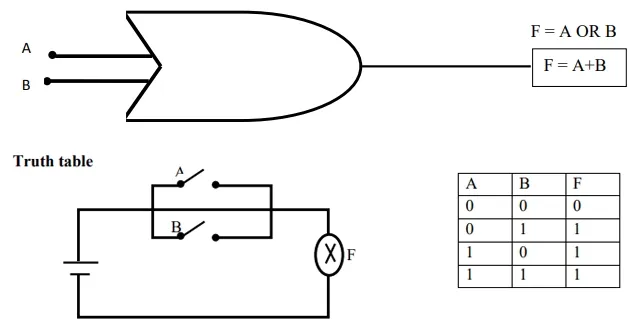Switch Arrangements equivalent to OR logic

#### THE EXCLUSIVE-OR (XOR) GATE

Exclusive-OR gate with only two inputs will give logic 1 output only if input A or input B is at logic1, but not when both A and B are at logic 1.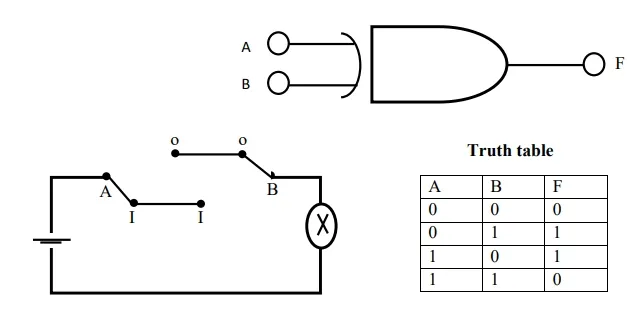#### THE NOT GATE

The NOT gate is a single input gate which gives an output that is the opposite of the input. For this reason it is sometimes called an INVERTER, a NEGATOR or a SIGN CHANGER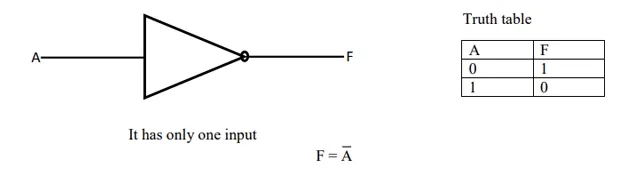#### THE NAND LOGIC GATE

The NAND gate is a NOT gate and an AND gate combined to form a NOT –AND gate (NAND) gate. The output of the NAND gate is the opposite of the AND gate.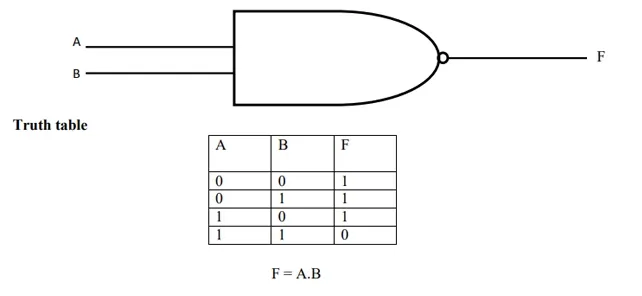THE NOR LOGIC GATE

The NOR gate is a NOT gate and an OR gate combined to form a NOT-OR (NOR) gate. The output of the NOR gate is the opposite of the OR gate.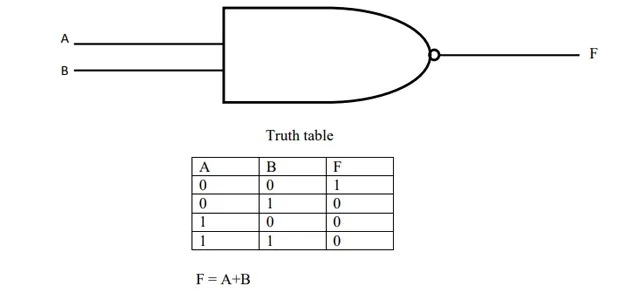### BUFFERS

A buffer has only one input and one output and its logical output is exactly the same as its input. Although the input and output voltage levels of buffer are identical, the currents present at the input and output can be very different.

#### WASSCE MAY /JUNE 2006 PAST QUESTION AND ANSWERS

1)      Draw the

2)      Circuit symbol of a three – input AND gate

3)      Truth – table of the gate in (a) (i)

Input AND gate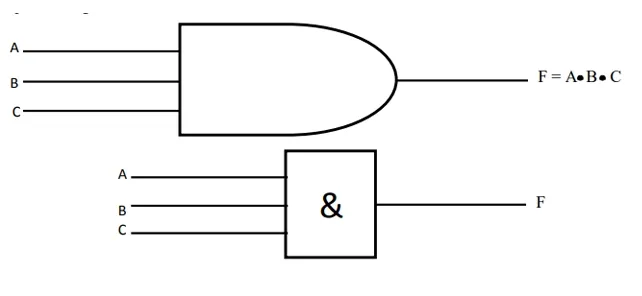F = A. B. C

Truth table

 A B C F 0 0 0 0 0 0 1 0 0 1 0 0 0 1 1 0 1 0 0 0 1 0 1 0 1 1 0 0 1 1 1 1

#### BOOLEAN IDENTITIES

Boolean Identity is a system of rules used to reduce complex calculation to simple expressions.

OR GATE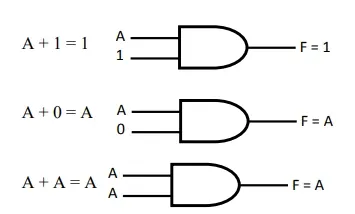SOLVED QUESTIONS

(1) Using switches draw a 3 input

(a) OR logic gate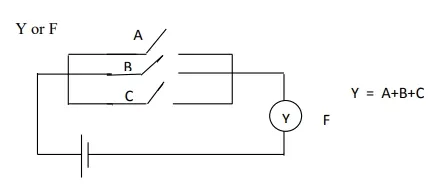(b) And logic gate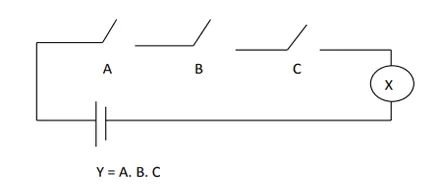(2) Using 2INPUT NAND, AND and OR logic gate only, draw a logic diagram for the Boolean  equation  A . B + AC  =  Y

Note A.B = AB Y = AB + AC

i.e.  AB is AND gate and AC is also AND passing through OR gate

Y = AB + AC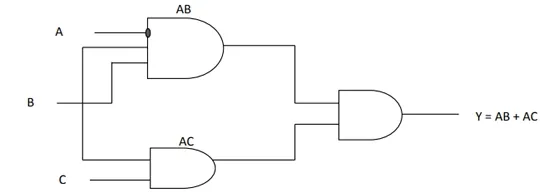(3) Draw a truth table for the Boolean expression

B. C + A = Z

 A B C B.C B.C + A 0 0 0 0 0 0 0 1 0 0 0 1 0 0 0 0 1 1 1 1 1 0 0 0 1 1 0 1 0 1 1 1 0 0 1 1 1 1 1 1

Writing Boolean expression from a figure / diagram

Y = (A . B)  +  ( B.  C)

2.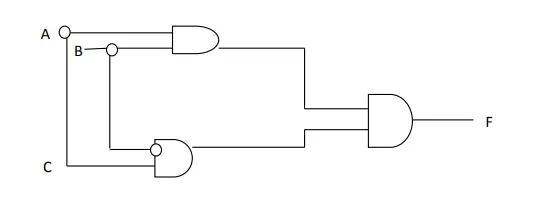1. Draw the logic circuit shown above

2. Indicate the output of each logic gate on the diagram

3. Write the Boolean expression

Solution

1.      Re – draw the logic circuit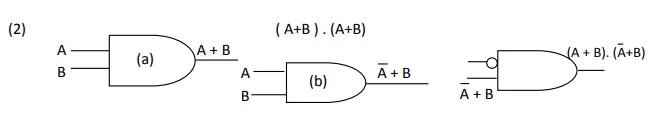Trial Test

1.      A voltage signal which changes smoothly and progressively between two extremes is called

(a) a logical waveform                           (b) an analogue waveform

(c) an interference                                   (d) a digital waveform

2.      Draw a truth table for the Boolean expression

(I)                 A . ( A + B ) = Y

(II)               A + A . B = Y

(III)            (A + B ) . ( A + C )

3.      Draw a network to generate the function

(i)    F = A . B + C

(ii)  F = A . B + A . C

(iii). F = ( A + B ) . ( A + B )

(iv).  F = A . B  +  B . A